# Dr. Foolish's Data Pies

#### Difficulty: MediumBelow are 8 data pies. Each pie has 2 pieces: a yellow one & a purple one. (If you're colorblind, then the purple piece will look dark gray to you while the yellow piece will appear light gray.) Your goal is to measure the angle of each pie piece. You could use a protractor, but there's a numerical way! Observe the formulae below:

## x = percentage number

You must divide x by 100 because the term percentage means "out of 100 pieces". To explain further for example, 55% = 0.55 or 55/100 or 11/20 in simplest terms.

After calculating the angle of 1 of the 2 pie pieces, consider this 2nd formula:

## [Angle of Other Pie Piece] = 360° - [Angle of 1st Pie Piece]

Because the 2 pie pieces have explementary angles of each other!

## Here come the data pies!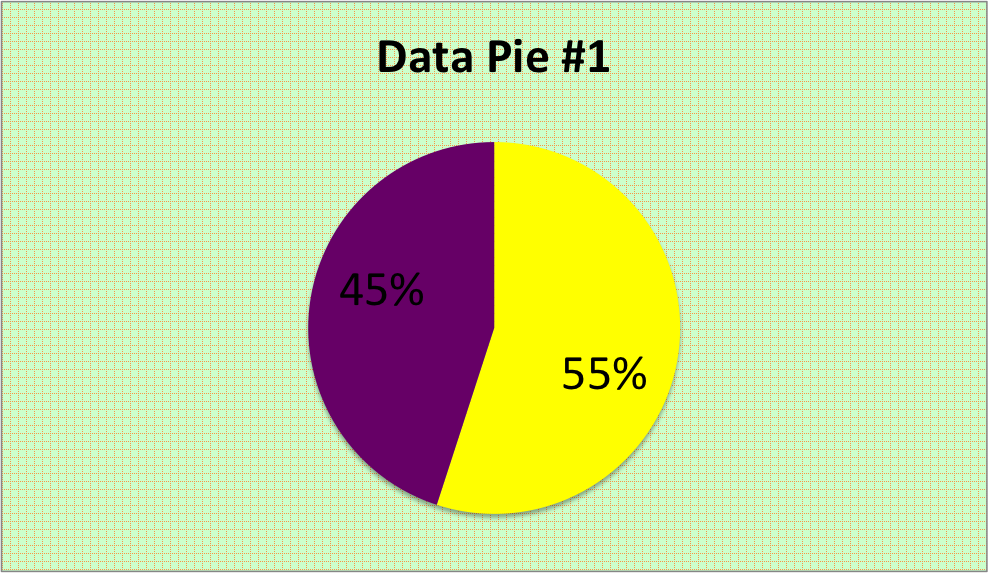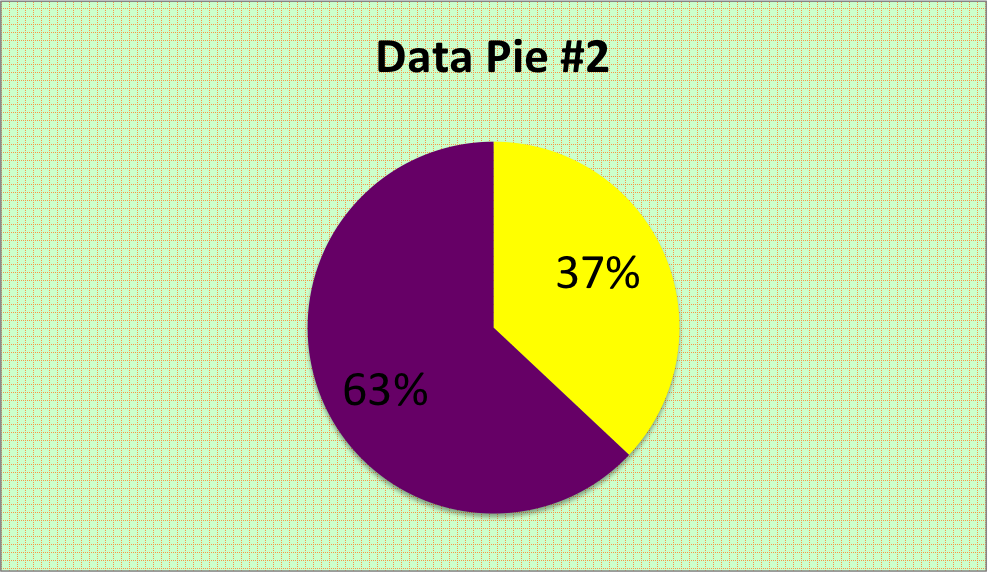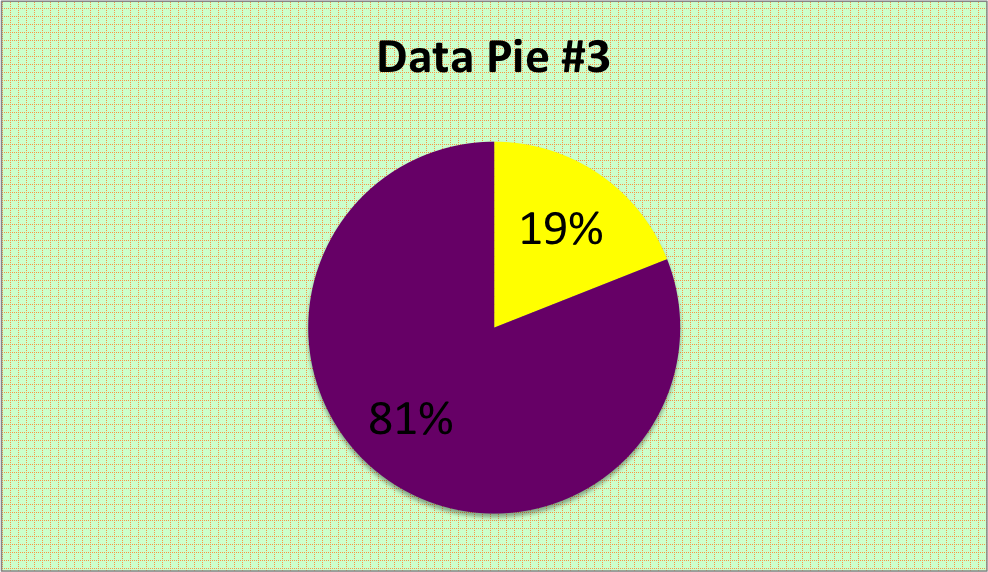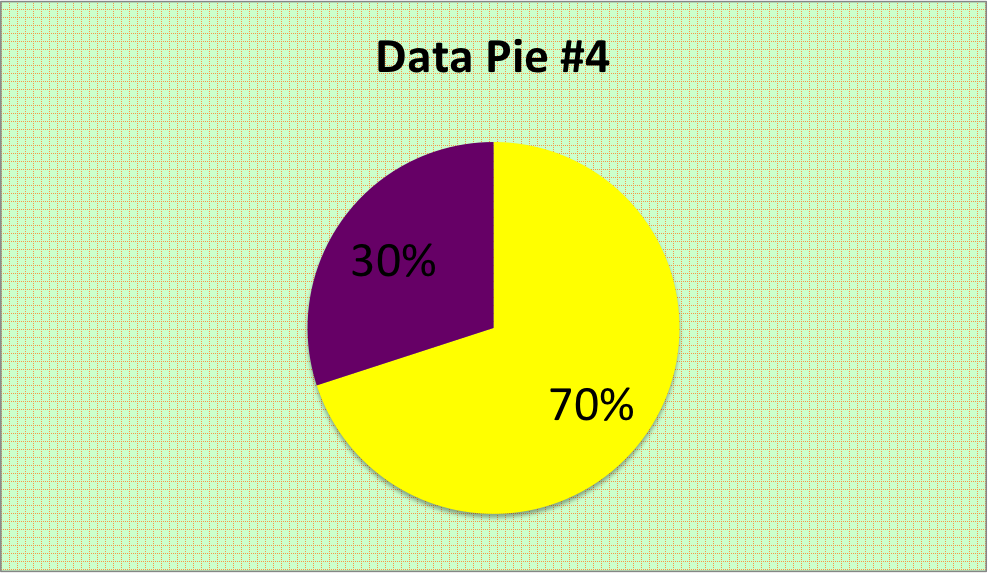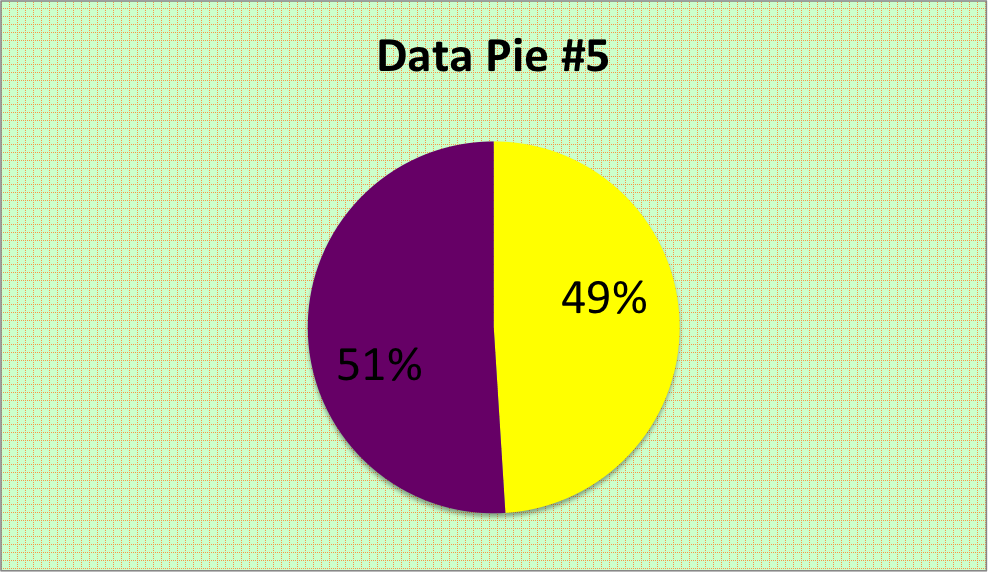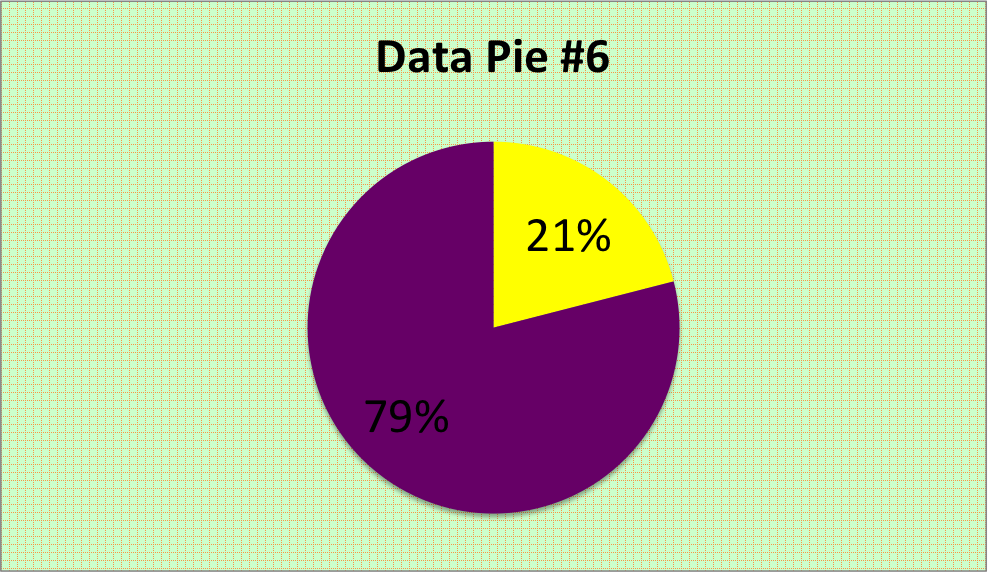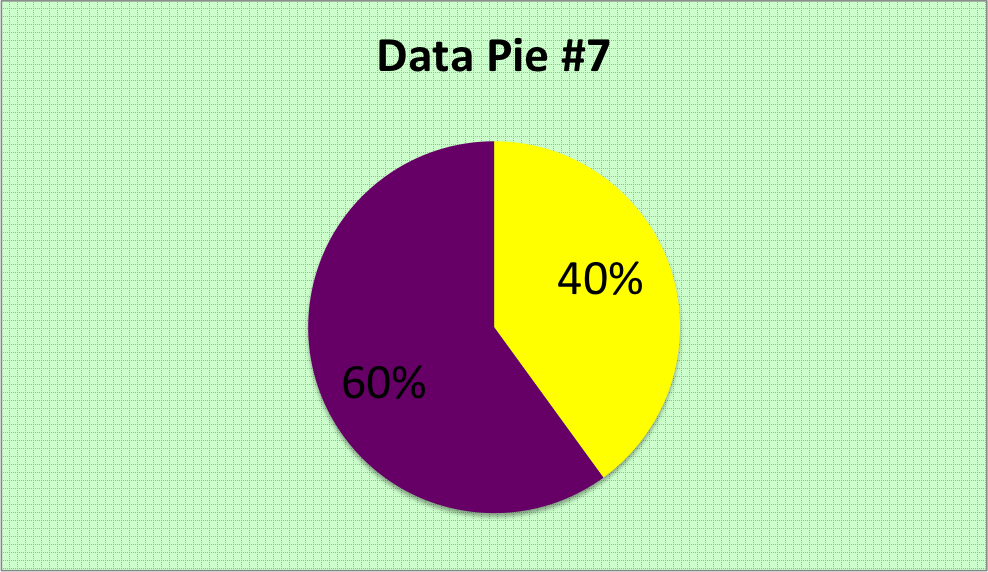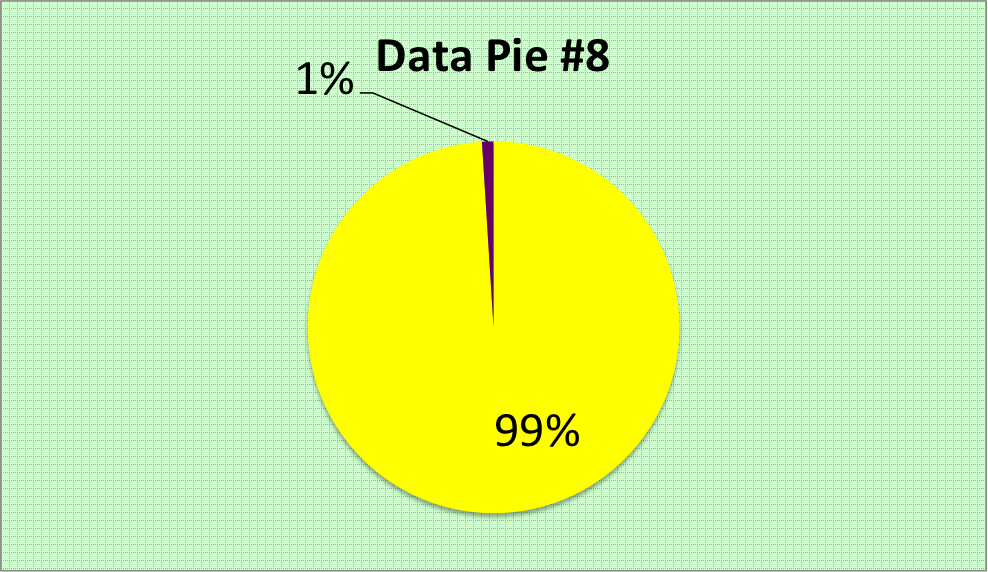Remember to plug the percentage number into x, then divide it by 100, then multiply that by 360.